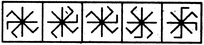# Non Verbal Reasoning - Classification - Discussion

### Discussion :: Classification - Section 1 (Q.No.9)

In each problem, out of the five figures marked (1), (2), (3), (4) and (5), four are similar in a certain manner. However, one figure is not like the other four. Choose the figure which is different from the rest.

9.

Choose the figure which is different from the rest.(1)     (2)     (3)     (4)     (5)

 [A]. 1 [B]. 2 [C]. 3 [D]. 4 [E]. 5

Explanation:

Figure (1) and fig. (3) can be rotated into each other and fig. (4) and fig. (5) can be rotated into each other.

 R. Kirthi Kumar said: (Dec 20, 2010) yaar i have not understood this , can somebody help me

 Seif El Din said: (Sep 9, 2011) After each pin's looking in the same direction you will find a single straight pin but in 2 you will find 3 straight pins. I dont see it related with rotation's.

 Fathima said: (Jun 3, 2013) In option (1) there is a + sign and multiply symbol passing through center point. In op (3) +sign and multiply symbol is rotated anti clockwise direction (or opposite of + and *). In (4) and (5) options. Symbol is rotated 135 degree anti clockwise direction. So option (2) is unrelated.

 Venu said: (Aug 27, 2015) Move each pin in the 1st figure and in anti clock wise direction, keep every pin in 4th position and you get 3rd figure. For 4th and 5th do the same but now this time in clock wise in 3rd position and you get 5th figure from 4th, 2nd is a loner!

 Rutuja said: (Jan 4, 2017) I still don't understand this. Please someone help me.

 Hardik Patel said: (Jan 25, 2017) Figure 5 is right because of the only one 90 angle arrow extend & other all figure three arrow is extend in 90 angle.

 David Samuel said: (Feb 15, 2017) No, the Answer is wrong. Answer 4 is the right option as all the figure is rotating in a clockwise direction except fig4.

 Dharpreet Kaur said: (Feb 28, 2017) Option 4 is correct. As all the figures are rotating in a clockwise direction except 4.

 Anand Rao said: (Jul 27, 2017) Options 4 and 5 are rotating in CW direction whereas Options 1, 2 and 3 rotating in ACW direction. Hence, the above logic doesn't imply here.

 Brindha.H said: (Sep 18, 2017) If the puzzle is odd man out. Then Fig. 4 is the answer. The middle parallel line is plain, others having one small "L" share is there.

 Punitha said: (Sep 26, 2017) If the puzzle is odd man out. Then Fig. 4 is the answer. The middle parallel line is plain, others having one small "L" the share is there.

 Tekpant said: (Aug 13, 2019) LLL following l in option A. LLL following lll in option B --> (so this is correct). LLL following l in option C. LLL following l in option D. LLL following l in option E.

 Mahesh said: (Feb 8, 2021) B is correct why because except 2 remaining all have 1L after 3 Continues L's.

 Shrikant said: (Jun 6, 2021) B is right. Imagine a straight line is dividing the objects. The part where there is only one L is similar in all except 2 in which L is facing outward. So the correct answer is 2 i.e. B

 Vamsi said: (Oct 14, 2021) I think 5 is the answer as it has the middle line cross while others having horizontal and vertical.